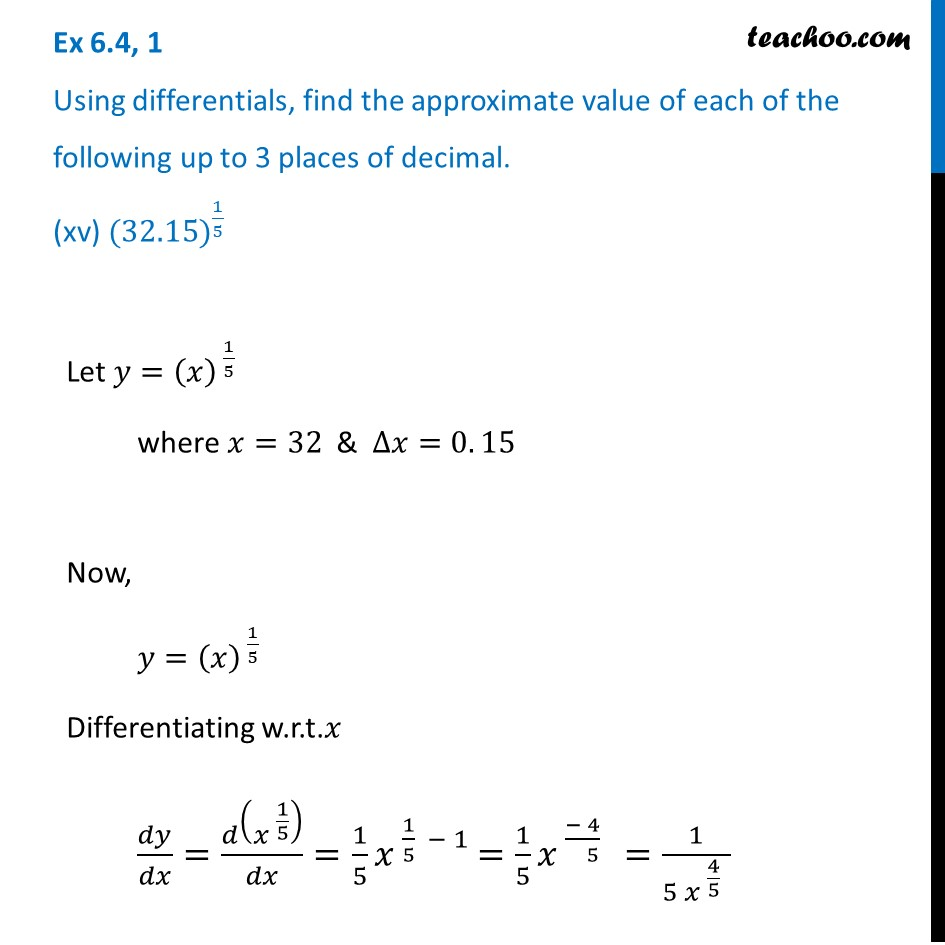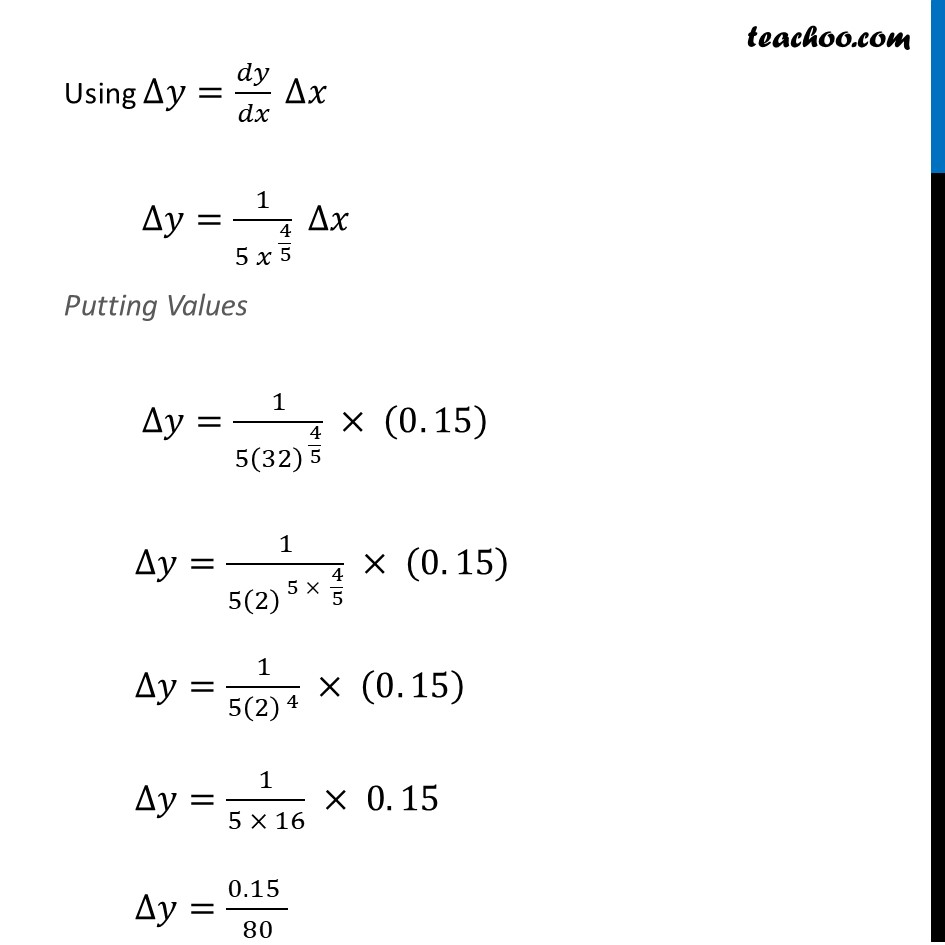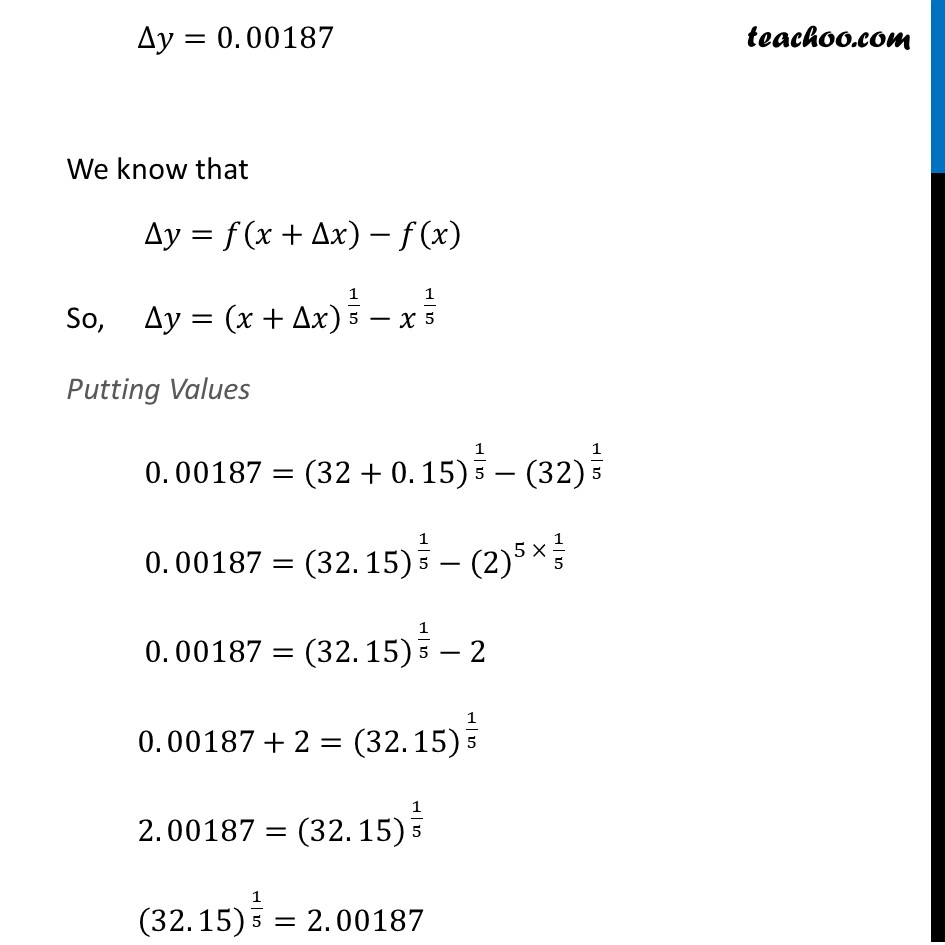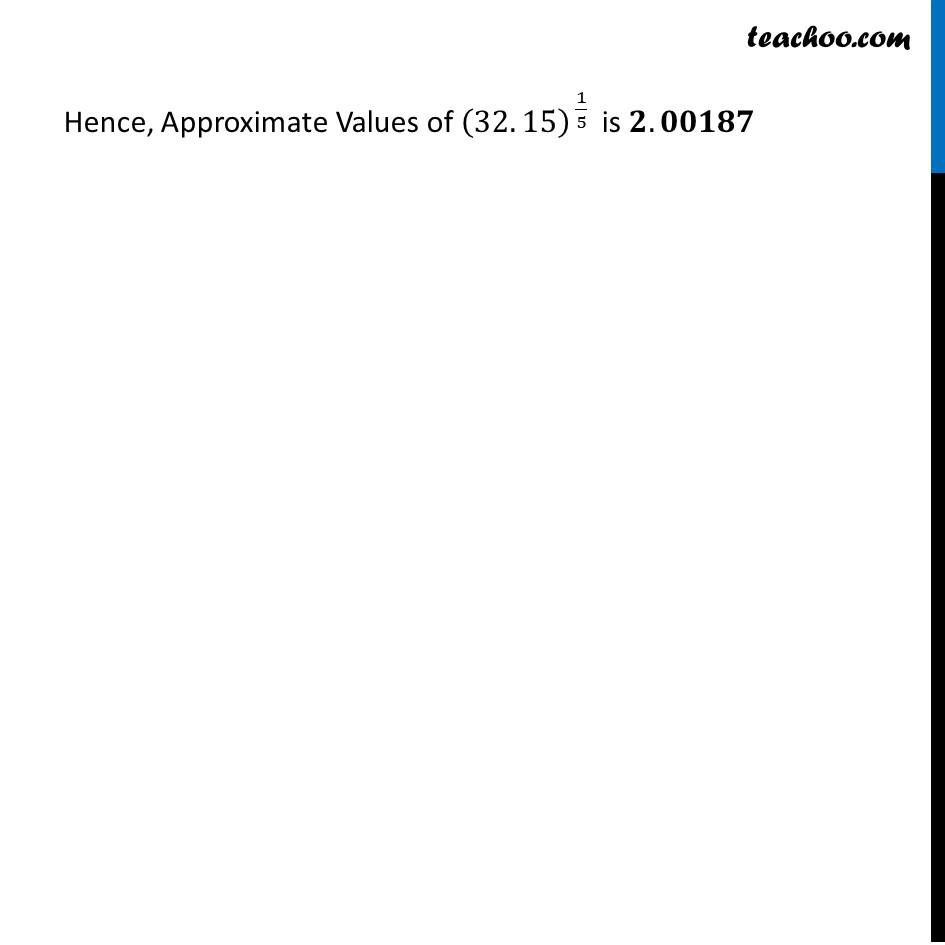Approximations (using Differentiation)

Chapter 6 Class 12 Application of Derivatives
Serial order wiseLearn in your speed, with individual attention - Teachoo Maths 1-on-1 Class

### Transcript

Question 1 Using differentials, find the approximate value of each of the following up to 3 places of decimal. (xv) 〖(32.15)〗^(1/5)Let 𝑦=(𝑥)^( 1/5) where 𝑥=32 & ∆𝑥=0. 15 Now, 𝑦=(𝑥)^( 1/5) Differentiating w.r.t.𝑥 𝑑𝑦/𝑑𝑥=𝑑(𝑥^( 1/5) )/𝑑𝑥=1/5 𝑥^( 1/5 − 1) =1/5 𝑥^( (− 4)/( 5) )=1/(5〖 𝑥〗^( 4/5 ) ) Using ∆𝑦=𝑑𝑦/𝑑𝑥 ∆𝑥 ∆𝑦=1/(5 〖𝑥 〗^(4/5) ) ∆𝑥 Putting Values ∆𝑦=1/(5(32)^( 4/5) ) × (0. 15) ∆𝑦=1/(5(2)^( 5 × 4/5) ) × (0. 15) ∆𝑦=1/(5(2)^( 4) ) × (0. 15) ∆𝑦=1/(5 × 16) × 0. 15 ∆𝑦=(0. 15" " )/80 ∆𝑦=0. 00187 We know that ∆𝑦=𝑓(𝑥+∆𝑥)−𝑓(𝑥) So, ∆𝑦=(𝑥+∆𝑥)^( 1/5)−〖𝑥 〗^(1/5) Putting Values 0. 00187=(32+0. 15)^( 1/5)−(32)^( 1/5) 0. 00187=(32. 15)^( 1/5)−(2)^(5 × 1/5 ) 0. 00187=(32. 15)^( 1/5)−2 0. 00187+2=(32. 15)^( 1/5) 2. 00187=(32. 15)^( 1/5) (32. 15)^( 1/5)=2. 00187 Hence, Approximate Values of (32. 15)^( 1/5) is 𝟐. 𝟎𝟎𝟏𝟖𝟕 Hence, Approximate Values of (32. 15)^( 1/5) is 𝟐. 𝟎𝟎𝟏𝟖𝟕